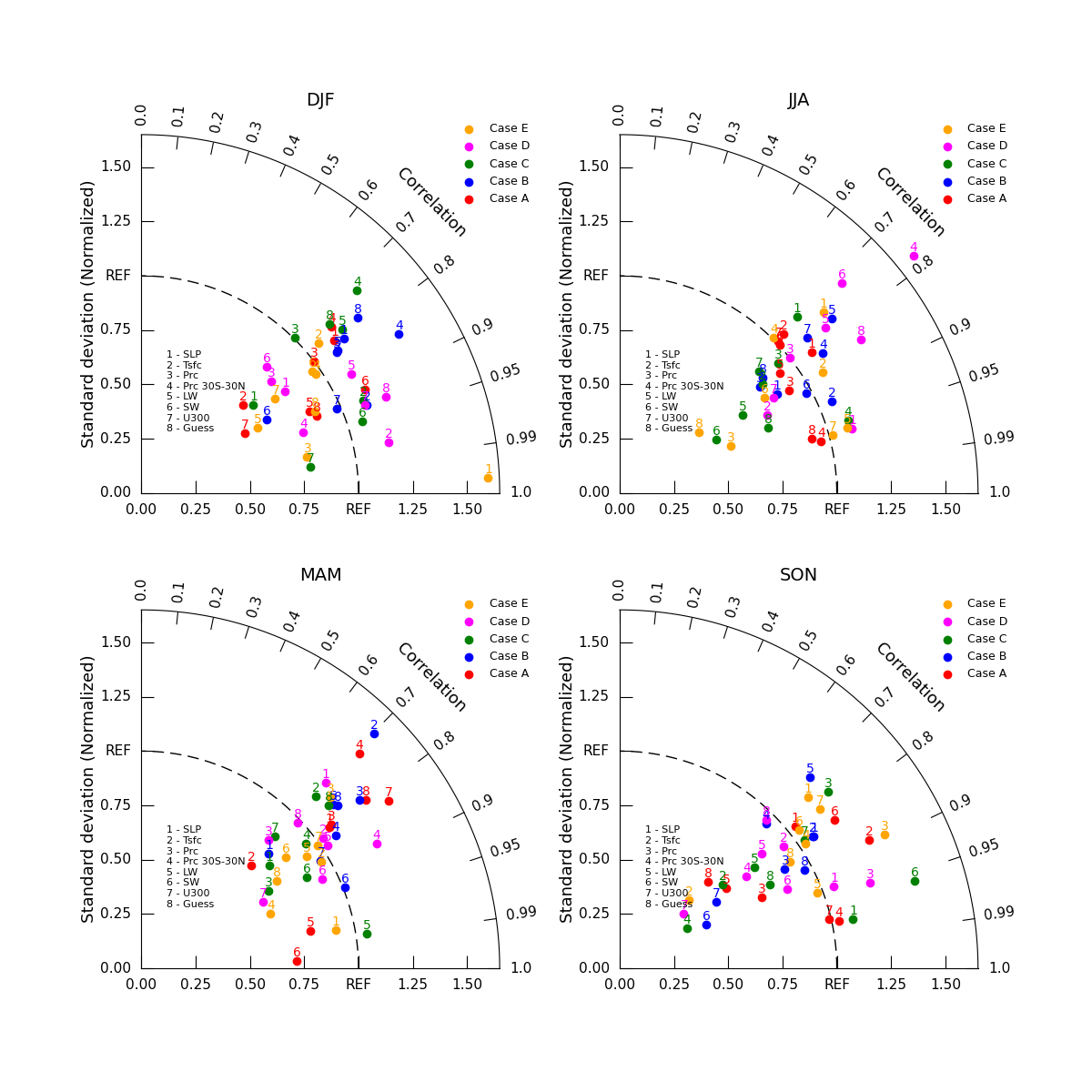# NCL_taylor_6.py#

This script illustrates the following concepts:
See following URLs to see the reproduced NCL plot & script:
Note: Due to limitations of matplotlib’s axisartist toolkit, we cannot include minor tick marks

between 0.9 and 0.99, as seen in the original NCL plot.

Import packages:

```import matplotlib.pyplot as plt
import numpy as np

import geocat.viz as gv
```

Plot:

```# Create figure
fig = plt.figure(figsize=(12, 12))

# Create a list of model names
namearr = ["SLP", "Tsfc", "Prc", "Prc 30S-30N", "LW", "SW", "U300", "Guess"]
nModel = len(namearr)

# Create a list of case names
casearr = ["Case A", "Case B", "Case C", "Case D", "Case E"]
nCase = len(casearr)

# Create lists of colors, labels, and main titles
colors = ["red", "blue", "green", "magenta", "orange"]
labels = ["Case A", "Case B", "Case C", "Case D", "Case E"]
maintitles = ["DJF", "JJA", "MAM", "SON"]

# Generate one plot for each season
for i in range(4):
# Create dummy data for the season
stddev = np.random.normal(1, 0.25, (nCase, nModel))
corrcoef = np.random.uniform(0.7, 1, (nCase, nModel))

# Create taylor diagram
da = gv.TaylorDiagram(fig=fig, rect=221 + i, label='REF')

# Add models case by case
for j in range(stddev.shape):
corrcoef[j],
xytext=(-4, 5),
fontsize=10,
color=colors[j],
label=labels[j],
marker='o')